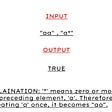# Encryption Hackerrank Problem

Task :- Given a string s, print it’s encrypted form. The encrypted form is formed by writing the string in a matrix form where

And then writing the string column-wise.

L -> length of string

For example:- string s = ‘ if man was meant to stay on the ground god would have given us roots ‘ then the matrix would look like :

And the answer would be “ imtgdvs fearwer mayoogo anouuio ntnnlvt wttddes aohghn sseoau “

Brute Force approach :- We can form a matrix of required length and then write the string in it row-wise. Print the characters while traversing column-wise.

The time complexity would be O(N) where N is length of string and space complexity is also O(N) as we are creating a matrix which stores this string.

Brute Force Code :-

Efficient Approach :- We can do this without forming the matrix by just traversing the string by incrementing the pointer by a certain step value(which is the ceil value of root of N).

For example in the above example we see N=54 and step value = ceil( sqrt ( 54 ) ) = 8

So we start with a letter and increment by step size till it is possible and print that letter, we do this for first step value letters. Before starting a new iteration, we print space(“ “) as required.

The time complexity is linear(O(N)) and space complexity is constant O(1).

Efficient Code :-

--

--

--

Love podcasts or audiobooks? Learn on the go with our new app.## More from Medium